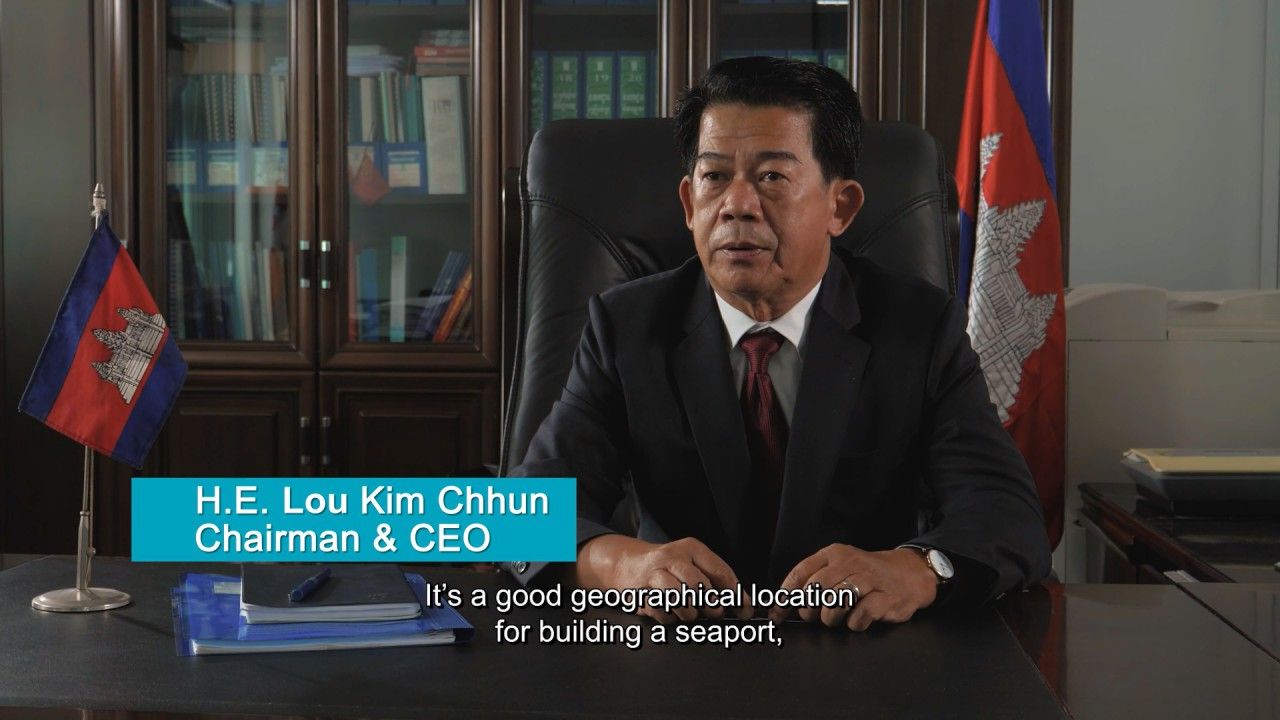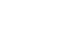Interim Financial Information For The Nine Month Period Ended 30 September 2018

In the third quarter of 2018, the total revenue of PAS is KHR 70,756,114,000 which increases by KHR 10,362,432,000 equal to 17.16% and the net profit is KHR 20,195,524,000 which increases by KHR 4,389,020,000 equal to 27.77% if compared to the same period of 2017. At the end of September 2018, total revenue is KHR 200,134,326,000 which increases by KHR 35,579,491,000 equal to 21.62% and net profit 29,518,958,000 which increase by 16,003,909,000 equal to 118.42%. At the end of September 2018 total asset is 1,303,087,168,000 which increase by 22,577,130,000 equal to 1.76% and total equity is KHR 663,849,370,000 which increases by KHR 17,238,816,000 equal to 2.67% ...

Interim Financial Information For The Nine Month Period Ended 30 September 2018

In the third quarter of 2018, the total revenue of PAS is KHR 70,756,114,000 which increases by KHR 10,362,432,000 equal to 17.16% and the net profit is KHR 20,195,524,000 which increases by KHR 4,389,020,000 equal to 27.77% if compared to the same period of 2017. At the end of September 2018, total revenue is KHR 200,134,326,000 which increases by KHR 35,579,491,000 equal to 21.62% and net profit 29,518,958,000 which increase by 16,003,909,000 equal to 118.42%. At the end of September 2018 total asset is 1,303,087,168,000 which increase by 22,577,130,000 equal to 1.76% and total equity is KHR 663,849,370,000 which increases by KHR 17,238,816,000 equal to 2.67%.